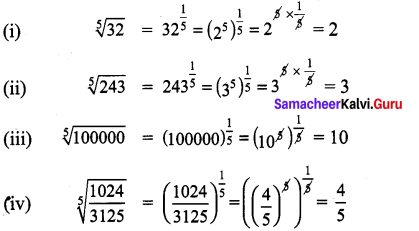## Tamilnadu Samacheer Kalvi 9th Maths Solutions Chapter 2 Real Numbers Ex 2.5

Question 1.
Write the following in the form of 5n:
(i) 625
(ii) $$\frac { 1 }{ 5 }$$
(iii) $$\sqrt { 5 }$$
(iv) $$\sqrt { 125 }$$
Solution:
(i) 625 = 54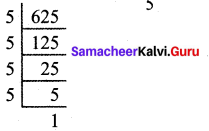(ii) $$\frac { 1 }{ 5 }$$ = 5-1

(iii) $$\sqrt { 5 }$$ = 5$$\frac { 1 }{ 2 }$$

(iv) $$\sqrt { 125 }$$
$$=\sqrt{5^{3}}=\left(5^{3}\right)^{\frac{1}{2}}=5^{\frac{3}{2}}$$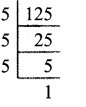Question 2.
Write the following in the form of 4n:
(i) 16
(ii) 8
(iii) 32
Solution:
(i) 16 = 42
(ii) 8 = $$4^{1} \times 4^{\frac{1}{2}}=4^{1+\frac{1}{2}}=4^{\frac{3}{2}}$$
(iii) 32 = $$4 \times 4 \times 4^{\frac{1}{2}}=4^{2+\frac{1}{2}}=4^{\frac{5}{2}}$$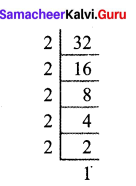Question 3.
Find the value of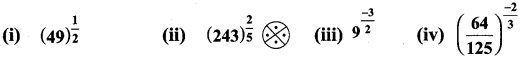Solution: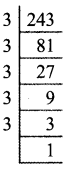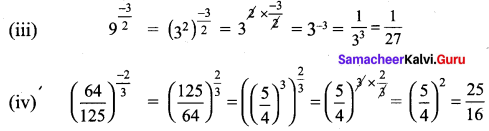Question 4.
Use a fractional index to write:
(i) $$\sqrt { 5 }$$
(ii) $$\sqrt{7}$$
(iii) ($$\sqrt{49}$$)5
((iv) $$\left(\frac{1}{\sqrt{100}}\right)^{7}$$
Solution: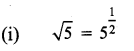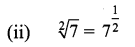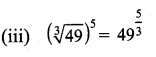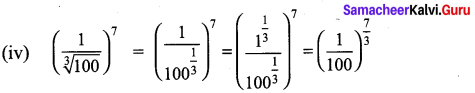Question 5.
Find the 5th root of
(i) 32
(ii) 243
(iii) 100000
(iv) $$\frac{1024}{3125}$$
Solution: